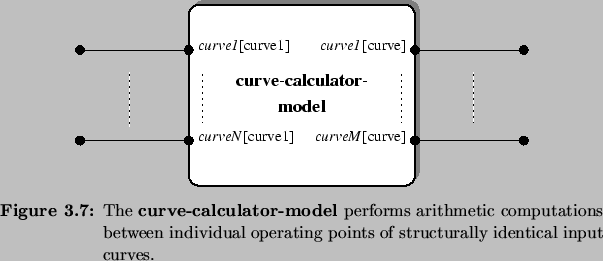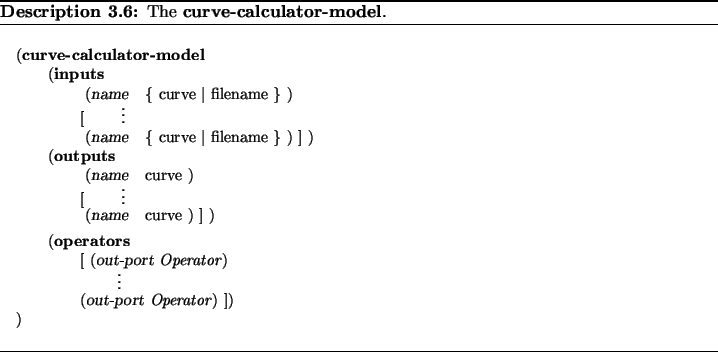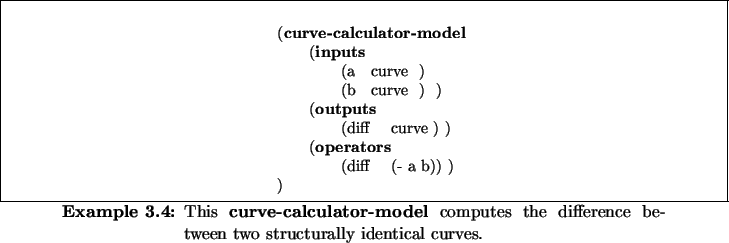Next: 3.6.3 The Curve-To-File-Model Up: 3.6 Auxiliary Models Previous: 3.6.1 The Arithmetic-Model

## 3.6.2 The Curve-Calculator-Model

The curve-calculator-model (Figure 3.7, Description 3.6) is able to perform arithmetic operations between corresponding operating points of structurally identical input curves, similar to the arithmetic-model. Example 3.4 demonstrates this behavior. The operator diff computes the difference of each curve response which exists in the curve at input port a as well as in the curve at input port b. The resulting curve, which can be considered the difference between the two input curves, is delivered at the output port named diff. Unless the structures of the involved curves are not identical SIESTA issues an error message.Rudi Strasser
1999-05-27